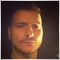# Why is this out of range????1067

```for(int i=0;i<rates_total;i++)
{
double Buffer=iCustom(_Symbol,_Period,"SuperTrendValues",0,i+1);
double Buffer_1=iCustom(_Symbol,_Period,"SuperTrendValues",0,i+2);

if(Buffer>Buffer_1)
{
Blue_1=true;
Red_1=false;
}
if(Buffer<Buffer_1)
{
Blue_1=false;
Red_1=true;
}

if(Blue_1==true)
{
TrendUp[i+1]=Buffer; // <--- ARRAY OUT OF RANGE
}
if(Red_1==true)
{
TrendDown[i+1]=Buffer;
}
}

return(rates_total);```25025

Arthur Singer:

go up to <rates_total-11067

Thank you very much!24261

`         TrendDown[i+1]=Buffer;`

How to do your lookbacks correctly #9 - #14 & #19